# Search Results (29)

View
Selected filters:
• CCSS.Math.Content.7.G.A.2Rating

This lesson unit is intended to help assess how well students are able to interpret and use scale drawings to plan a garden layout. This involves using proportional reasoning and metric units.

Subject:
Algebra
Geometry
Ratios and Proportions
Material Type:
Assessment
Lesson Plan
Provider:
Shell Center for Mathematical Education
U.C. Berkeley
Provider Set:
Mathematics Assessment Project (MAP)
Mathematics Assessment Project (MAP)
04/26/2013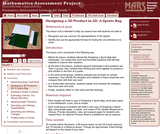Rating

This lesson unit is intended to help you assess how well students are able to: recognize and use common 2D representations of 3D objects and identify and use the appropriate formula for finding the circumference of a circle.

Subject:
Education
Mathematics
Geometry
Material Type:
Assessment
Lesson Plan
Provider:
Florida Center for Research in Science, Technology, Engineering, and Mathematics
Shell Center for Mathematical Education
U.C. Berkeley
Provider Set:
iCPALMS: A Standards-based K-12 Resources and Tools Pathway
Mathematics Assessment Project (MAP)
Mathematics Assessment Project (MAP)
Author:
http://map.mathshell.org/
04/26/2013Rating

This lesson unit is intended to help you assess how well students are able to use geometric properties to solve problems. In particular, it will support you in identifying and helping students who have the following difficulties: Solving problems relating to using the measures of the interior angles of polygons; and solving problems relating to using the measures of the exterior angles of polygons.

Subject:
Geometry
Material Type:
Assessment
Lesson Plan
Provider:
Shell Center for Mathematical Education
U.C. Berkeley
Provider Set:
Mathematics Assessment Project (MAP)
Mathematics Assessment Project (MAP)
04/26/2013Rating

This lesson unit is intended to help you assess how well students are able to: solve simple problems involving ratio and direct proportion; choose an appropriate sampling method; and collect discrete data and record them using a frequency table.

Subject:
Education
Geometry
Measurement and Data
Ratios and Proportions
Material Type:
Assessment
Lecture Notes
Lesson Plan
Teaching/Learning Strategy
Provider:
Florida Center for Research in Science, Technology, Engineering, and Mathematics
Shell Center for Mathematical Education
U.C. Berkeley
Provider Set:
iCPALMS: A Standards-based K-12 Resources and Tools Pathway
Mathematics Assessment Project (MAP)
Mathematics Assessment Project (MAP)
Author:
http://map.mathshell.org/
04/26/2013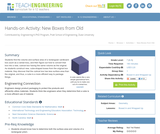Conditions of Use:
Rating

Students find the volume and surface area of a rectangular box (e.g., a cereal box), and then figure out how to convert that box into a new, cubical box having the same volume as the original. As they construct the new, cube-shaped box from the original box material, students discover that the cubical box has less surface area than the original, and thus, a cube is a more efficient way to package things.

Subject:
Engineering
Geometry
Material Type:
Activity/Lab
Lesson Plan
Provider:
TeachEngineering
Provider Set:
TeachEngineering
Author:
Mary R. Hebrank
01/20/2009Rating

This lesson unit is intended to help you assess how well students are able to: Model a situation; make sensible, realistic assumptions and estimates; and use assumptions and estimates to create a chain of reasoning, in order to solve a practical problem.

Subject:
Geometry
Material Type:
Assessment
Lesson Plan
Provider:
Shell Center for Mathematical Education
U.C. Berkeley
Provider Set:
Mathematics Assessment Project (MAP)
Mathematics Assessment Project (MAP)
04/26/2013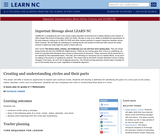Conditions of Use:
Remix and Share
Rating

This lesson will offer a hands-on opportunity to explore and construct circles. Students will develop a definition for identifying the parts of a circle such as the center, radius, diameter, chord, and circumference. Students will use compasses and rulers in constructing these parts of a circle.

Subject:
Geometry
Material Type:
Lesson Plan
Provider:
University of North Carolina at Chapel Hill School of Education
Provider Set:
LEARN NC Lesson Plans
Author:
Patricia Tingen
06/25/1999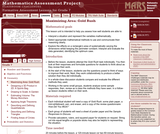Rating

This lesson unit is intended to help you assess how well students are able to: Interpret a situation and represent the variables mathematically; select appropriate mathematical methods to use; explore the effects on the area of a rectangle of systematically varying the dimensions whilst keeping the perimeter constant; interpret and evaluate the data generated and identify the optimum case; and communicate their reasoning clearly.

Subject:
Geometry
Material Type:
Assessment
Lesson Plan
Provider:
Shell Center for Mathematical Education
U.C. Berkeley
Provider Set:
Mathematics Assessment Project (MAP)
Mathematics Assessment Project (MAP)
04/26/2013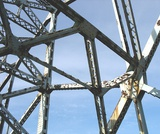Conditions of Use:
Remix and Share
Rating

Students learn to classify triangles by the size of the angles and the measures of the sides.Key ConceptsTriangles are polygons with three sides.Scalene triangles have all sides with a different length and all angles with a different measure.Isosceles triangles have two sides with the same length and two angles with the same measure.Equilateral triangles have all sides with the same length and all angles with the same measure.Acute triangles have all angles with a measure less than 90°.Obtuse triangles have one angle with a measure greater than 90°.Right triangles have one angle with a measure of 90°.ELL: Keep in mind that consistency at the beginning is very important as students begin to learn and apply math vocabulary.Goals and Learning ObjectivesExplore conditions that result in triangles.Identify types of triangles based on the measure of the angles or the measures of the sides.

Subject:
Geometry
Material Type:
Lesson Plan
Provider:
Pearson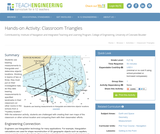Conditions of Use:
Rating

Students use bearing measurements to triangulate and determine objects' locations. Working in teams of two or three, they must put on their investigative hats as they take bearing measurements to specified landmarks in their classroom (or other rooms in the school) from a "mystery location." With the extension activity, students are challenged with creating their own maps of the classroom or other school location and comparing them with their classmates' efforts.

Subject:
Education
Material Type:
Activity/Lab
Provider:
TeachEngineering
Provider Set:
TeachEngineering
Author:
Janet Yowell
Malinda Schaefer Zarske
Matt Lippis
10/14/2015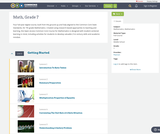Conditions of Use:
Remix and Share
Rating

Four full-year digital course, built from the ground up and fully-aligned to the Common Core State Standards, for 7th grade Mathematics. Created using research-based approaches to teaching and learning, the Open Access Common Core Course for Mathematics is designed with student-centered learning in mind, including activities for students to develop valuable 21st century skills and academic mindset.

Subject:
Mathematics
Material Type:
Full Course
Provider:
Pearson
10/06/2016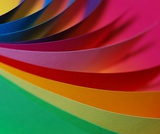Conditions of Use:
Remix and Share
Rating

Students discuss what they know about shapes and their characteristics through a paper-folding activity that results in a parallelogram.Key ConceptsQuadrilaterals and triangles are classified by their different characteristics; the types of angles and sides define the shapes. While students are familiar with some of the characteristics of these shapes, they begin to explore other aspects of theses figures. Students review what they know about these shapes so far.Goals and Learning ObjectivesReview characteristics that describe quadrilaterals and triangles.Discuss what students know about these shapes.Explore other aspects of these shapes.

Subject:
Geometry
Material Type:
Lesson Plan
Provider:
Pearson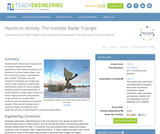Conditions of Use:
Rating

Students learn about radar imaging and its various military and civilian applications that include recognition and detection of human-made targets, and the monitoring of space, deforestation and oil spills. They learn how the concepts of similarity and scaling are used in radar imaging to create three-dimensional models of various targets. Students apply the critical attributes of similar figures to create scale models of a radar imaging scenario using infrared range sensors (to emulate radar functions) and toy airplanes (to emulate targets). They use technology tools to measure angles and distances, and relate the concept of similar figures to real-world applications.

Subject:
Education
Material Type:
Activity/Lab
Provider:
TeachEngineering
Provider Set:
TeachEngineering
Author:
Mounir Ben Ghalia
Rocio Denise Nava
10/14/2015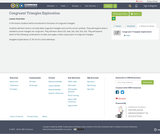Conditions of Use:
Remix and Share
Rating

In this lesson students will be introduced to the basics of congruent triangles.Students will learn how to correctly label congruent triangles and use the correct symbols. They will explore what is needed to prove triangles are congruent. They will learn about SSS, AAA, SAS, ASA, SSA, SAS. They will explore which of the following combinations of sides and angles create unique pairs of congruent triangles. Geogebra Explorations CC BY SA 4.0 Lorena Mendoza

Subject:
Mathematics
Material Type:
Lesson Plan
Author:
Julie Stoker
04/27/2018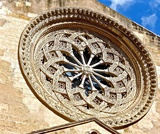Conditions of Use:
Remix and Share
Rating

Lesson OverviewStudents explore different polygons by drawing them, measuring angles, and manipulating interactive sketches to find the angle sum for any polygon. Students also explore the angle measures in regular polygons.Key ConceptsThe angle sum in a triangle is 180°. A quadrilateral can be composed of two triangles, so the angle sum of a quadrilateral is 360°.The number of triangles that compose a polygon is two less than the number of sides (angles). The sum of the interior angles in a polygon with n sides is 180° (n – 2).Goals and Learning ObjectivesFind angle sums in polygons.Generalize to find the angle sum for any polygon.Find interior angle measures for regular polygons.

Subject:
Geometry
Material Type:
Lesson Plan
Provider:
Pearson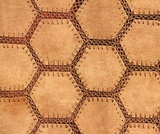Conditions of Use:
Remix and Share
Rating

Students learn more about the characteristics of parallelograms by folding paper and measuring the angles in a parallelogram. Students use a ruler and protractor to draw parallelograms with given properties. Then, students use a ruler and protractor to draw a rectangle.Key ConceptsOpposite angles of a parallelogram are congruent.Consecutive angles of a parallelogram are supplementary.Diagonals of a parallelogram bisect each other.Diagonals of a rectangle are congruent.Goals and Learning ObjectivesAccess prior knowledge of parallelograms.Understand that the sum of angle measures in any quadrilateral is 360°.Understand the relationship of the angles and diagonals in a parallelogram.Understand the relationship of the angles and diagonals in a rectangle.

Subject:
Geometry
Material Type:
Lesson Plan
Provider:
Pearson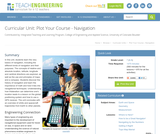Conditions of Use:
Rating

In this unit, students learn the very basics of navigation, including the different kinds of navigation and their purposes. The concepts of relative and absolute location, latitude, longitude and cardinal directions are explored, as well as the use and principles of maps and a compass. Students discover the history of navigation and learn the importance of math and how it ties into navigational techniques. Understanding how trilateration can determine one's location leads to a lesson on the global positioning system and how to use a GPS receiver. The unit concludes with an overview of orbits and spacecraft trajectories from Earth to other planets.

Subject:
Engineering
Physical Geography
Material Type:
Full Course
Provider:
TeachEngineering
Provider Set:
TeachEngineering
10/14/2015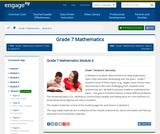Conditions of Use:
Remix and Share
Rating

In Module 6, students delve further into several geometry topics they have been developing over the years.  Grade 7 presents some of these topics, (e.g., angles, area, surface area, and volume) in the most challenging form students have experienced yet.  Module 6 assumes students understand the basics.  The goal is to build a fluency in these difficult problems.  The remaining topics, (i.e., working on constructing triangles and taking slices (or cross-sections) of three-dimensional figures) are new to students.

Subject:
Geometry
Material Type:
Module
Provider:
New York State Education Department
Provider Set:
EngageNY
02/02/2014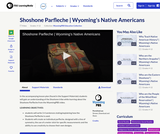Conditions of Use:
Remix and Share
Rating

In the accompanying lesson plan (found in the Support Materials) students will gain an understanding of the Shoshone tribe while learning about the Shoshone Parfleche from the WyomingPBS video.

LEARNING OBJECTIVES:

students will write 3-4 sentences stating/explaining how the Shoshone Parfleche is used.
Students will create an individual parfleche, designed with a line of symmetry, the use of a meter stick for specific measurements and the ability to use creativity to choose their own designs.

Subject:
English Language Arts
Mathematics
Geometry
Material Type:
Activity/Lab
Lesson
Provider:
Wyoming PBS
09/17/2019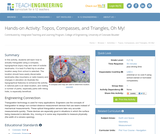Conditions of Use:
Rating

In this activity, students will learn how to actually triangulate using a compass, topographical (topo) map and view of outside landmarks. It is best if a field trip to another location away from school is selected. The location should have easily discernable landmarks (like mountains or radio towers) and changes in elevation (to illustrate the topographical features) to enhance the activity. A national park is an ideal location, and visiting a number of parks, especially parks with hiking trails, is especially beneficial.

Subject:
Education
Material Type:
Activity/Lab
Provider:
TeachEngineering
Provider Set:
TeachEngineering
Author:
Janet Yowell
Malinda Schaefer Zarske
Matt Lippis